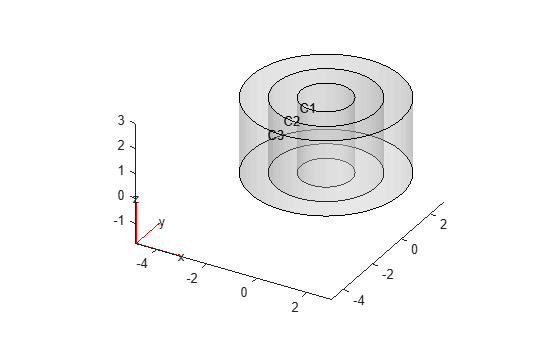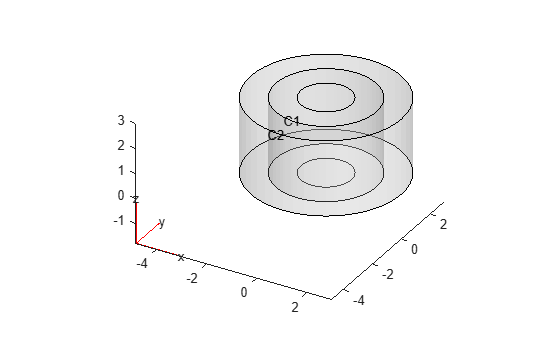# mergeCells

Merge geometry cells

Since R2023b

## Syntax

``h = mergeCells(g,cellIDs)``

## Description

example

````h = mergeCells(g,cellIDs)` merges two cells of the 3-D geometry `g` by removing their shared faces. `cellIDs` must be a vector of two cell IDs. The specified cells must share at least one face.`mergeCells` renumbers cells, faces, edges, and vertices in the resulting geometry. The merged cell gets the first of the specified cell IDs. The function decreases all cell IDs following the second of the specified cell IDs by 1. Because `mergeCells` removes shared faces, it also removes edges and vertices belonging only to these faces and decreases IDs of all subsequent faces, edges, and vertices.```

## Examples

collapse all

Merge two cells in a nested cylinder consisting of three cells.

Create a nested cylinder.

`gm = multicylinder([1 2 3],3);`

Plot the cylinder with cell labels.

`pdegplot(gm,CellLabels="on",FaceAlpha=0.5);`Merge the two inner cells.

`newgm = mergeCells(gm,[1 2]);`

Plot the resulting geometry. While `mergeCells` removes shared faces, it does not merge faces of the merged cells. So, cell 1 has two top and two bottom faces.

`pdegplot(newgm,CellLabels="on",FaceAlpha=0.5);`## Input Arguments

collapse all

Original multicell geometry, specified as an `fegeometry` object or `DiscreteGeometry` object.

IDs of two cells to merge, specified as a vector of two positive integers.

## Output Arguments

collapse all

Modified geometry, returned as an `fegeometry` object or a handle to a `DiscreteGeometry` object.

• If the original geometry `g` is an `fegeometry` object, then `h` is a new `fegeometry` object representing the modified geometry. The original geometry `g` remains unchanged. To replace the original geometry with the modified geometry, use the original geometry name as the output argument, for example, `g = mergeCells(g,[1 2])`.

• If the original geometry `g` is a `DiscreteGeometry` object, then `h` is a handle to the modified `DiscreteGeometry` object `g`.

## Tips

• While `mergeCells` removes shared faces of the merged cells, it does not merge faces of the merged cell. So, the resulting geometry can have extraneous faces. For example, if you merge two stacked cubes, `mergeCells` removes the face between the cubes,but each side of the merged cell consists of two faces, not one face, for a total of 10 faces in the merged geometry.

• Merging modifies a geometry, but it does not modify the mesh. After merging cells, call `generateMesh` for a proper mesh association with the new geometry.

## Version History

Introduced in R2023b